# Find a differential operator that annihilates the given function. xex -x sin 8x +x A differential...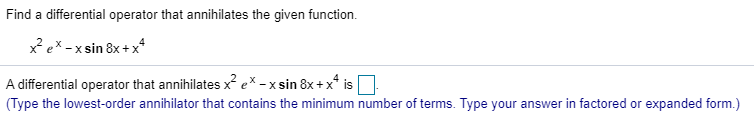Find a differential operator that annihilates the given function. xex -x sin 8x +x A differential operator that annihilates xe*- xsin 8x + x4 is (Type the lowest-order annihilator that contains the minimum number of terms. Type your answer in factored or expanded form.)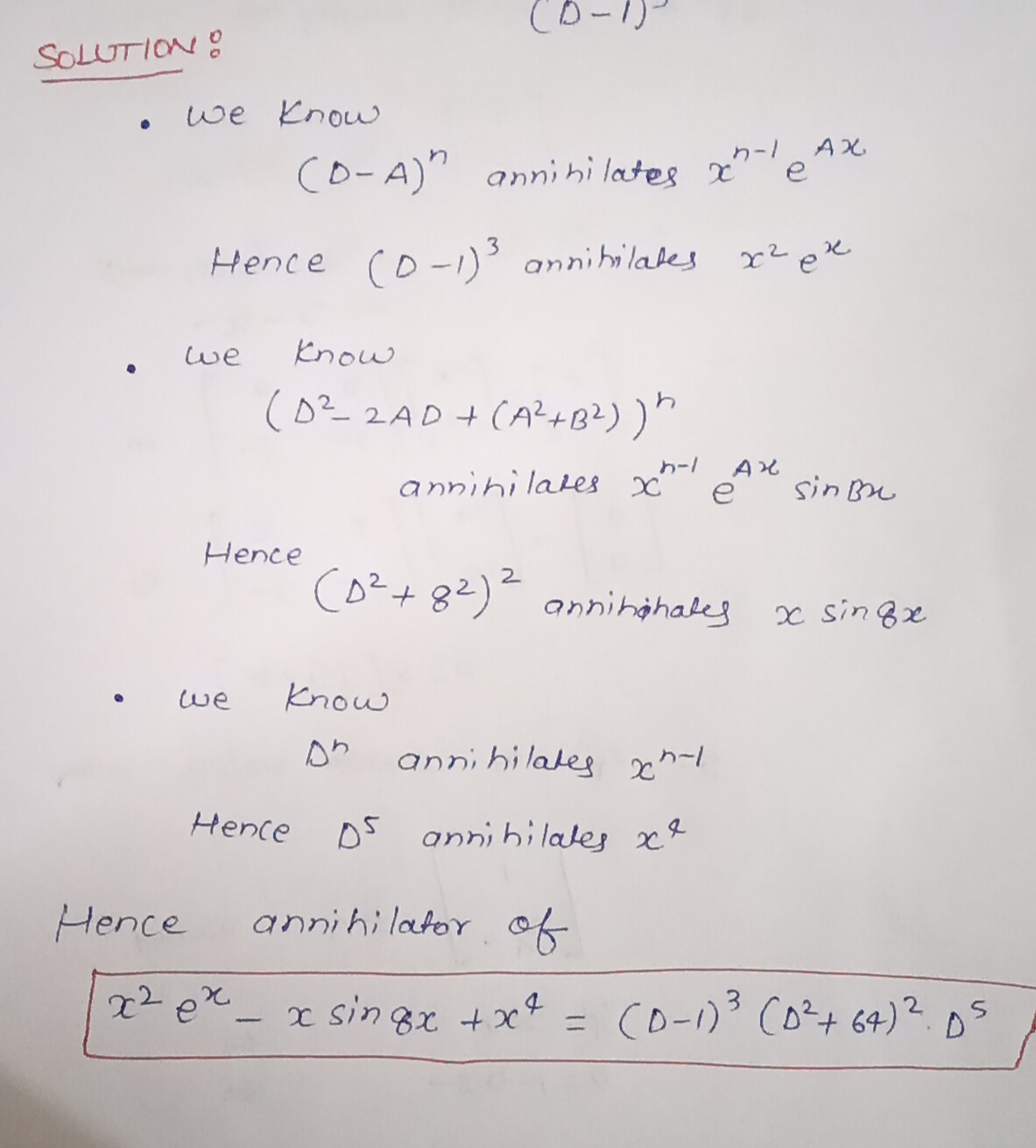##### Add Answer of: Find a differential operator that annihilates the given function. xex -x sin 8x +x A differential...
Similar Homework Help Questions
• ### Find a differential operator that annihilates the given function. Thank you! Find a differential operator that...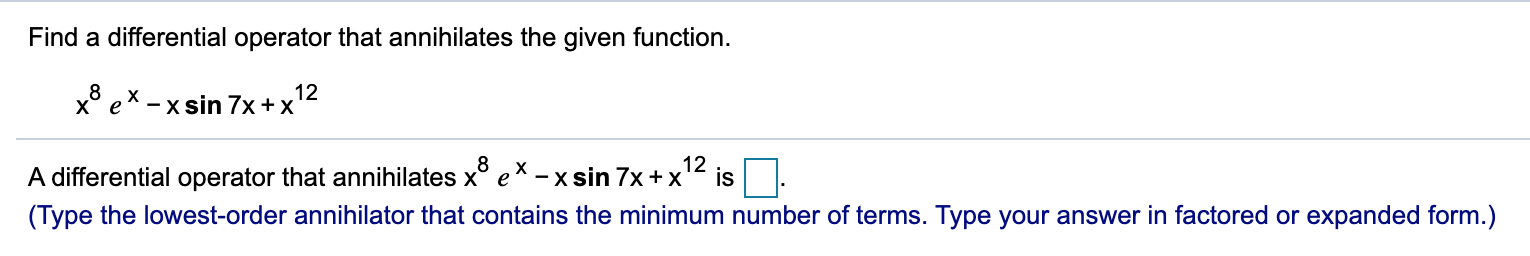Find a differential operator that annihilates the given function. Thank you! Find a differential operator that annihilates the given function. x8 ex - x sin 7x + x 12 12 A differential operator that annihilates x ex-xsin 7x + x is (Type the lowest-order annihilator that contains the minimum number of terms. Type your answer in factored or expanded form.)

• ### Find a differential operator that annihilates the given function. x8 ex - x sin 7x+x 12...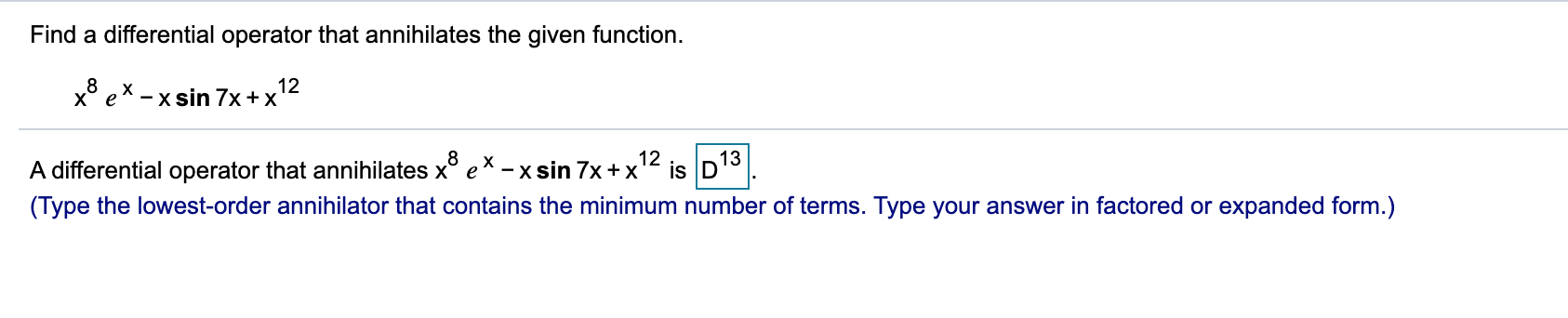Find a differential operator that annihilates the given function. x8 ex - x sin 7x+x 12 A differential operator that annihilates x ex - x sin 7x+x? 12 13 is D (Type the lowest-order annihilator that contains the minimum number of terms. Type your answer in factored or expanded form.)

• ### please answer alll! its greatly appreicated Find a general solution. u" + 33u = 0 u(t)...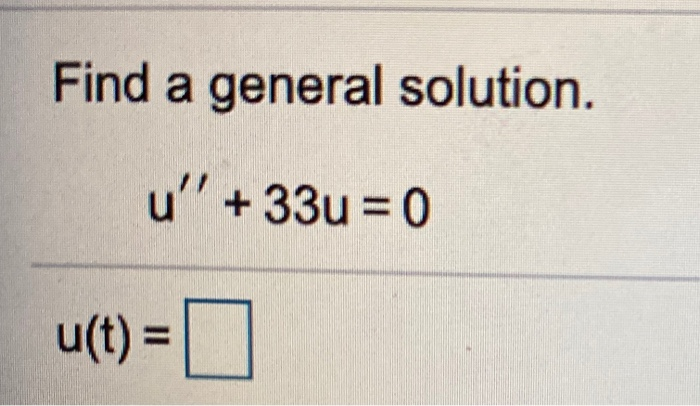please answer alll! its greatly appreicated Find a general solution. u" + 33u = 0 u(t) = [. Solve the given initial value problem. y"' + 2y' +10y = 0; y(0) = 4, y'(0) = - 3 y(t) = Use the annihilator method to determine the form of a particular solution for the given equation V'+w-20 = cos(-3x) + 11 Find a differential operator that will annihilate the nonhomogeneity cos ( - 3x) + 11. (Type the lowest-order annihilator that...

• ### find the differential operator that annihilates the given function x^(9)*e^(-3x)*sin(-12x)+x

find the differential operator that annihilates the given function x^(9)*e^(-3x)*sin(-12x)+x

• ### Find a linear differential operator that annihilates the given function Y= e^-x sin(x) +x+3xe^6x

Find a linear differential operator that annihilates the given function Y= e^-x sin(x) +x+3xe^6x

• ### QUESTION 2 Find a differential operator that annihilates the given function. xºe-3xsin (-12x) +X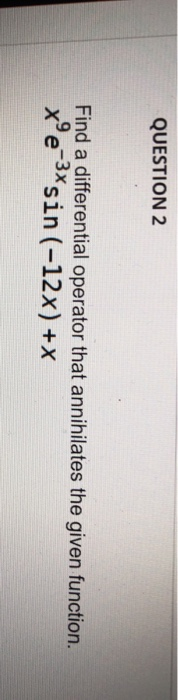QUESTION 2 Find a differential operator that annihilates the given function. xºe-3xsin (-12x) +X

• ### Find a differential operator that annihilates the given function. xe-3*sin(-12x) +X odlo-3)2 +144]1 08. [(0+3)2–144]1º oc[(0-3)2-144]1_4...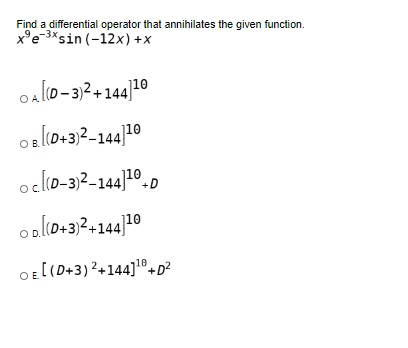Find a differential operator that annihilates the given function. xe-3*sin(-12x) +X odlo-3)2 +144]1 08. [(0+3)2–144]1º oc[(0-3)2-144]1_4 ool(D+3)2+144]10 OE[(D+3) 2+144] +02

• ### QUESTION 2 Find a differential operator that annihilates the given function. x'e 3xsin (-12x) +X [(0+3)2+144110...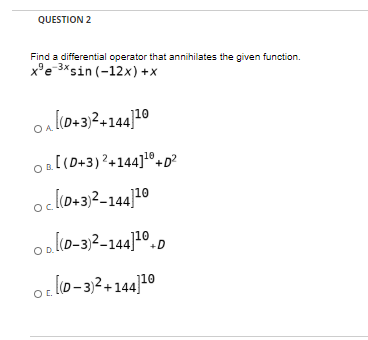QUESTION 2 Find a differential operator that annihilates the given function. x'e 3xsin (-12x) +X [(0+3)2+144110 [(0+3)2+144].0 +02 oc[(0+3)2-144]10 ook(0-3)2-144]20 of 10–3)2+144]10 D

• ### QUESTION 11 Find a differential operator that annihilates the given function. xºe-3xsin (-12x) +X A[(D+3) 2+144]+D2...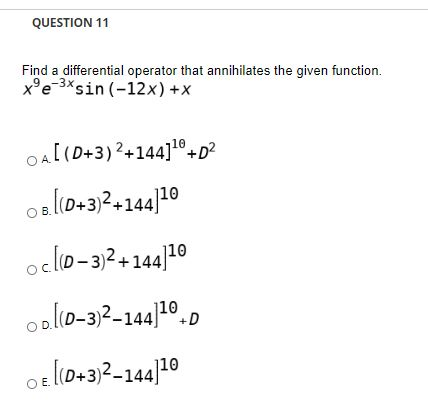QUESTION 11 Find a differential operator that annihilates the given function. xºe-3xsin (-12x) +X A[(D+3) 2+144]+D2 os (0+3)2+144]10 ocllo-3)2 +144]10 ool(D-3)2-144] 1070 Oe [(0+3)2-144]10

• ### Do just the marked ones (21,22,25) PART III. Given these pairs of differential operators, what operator...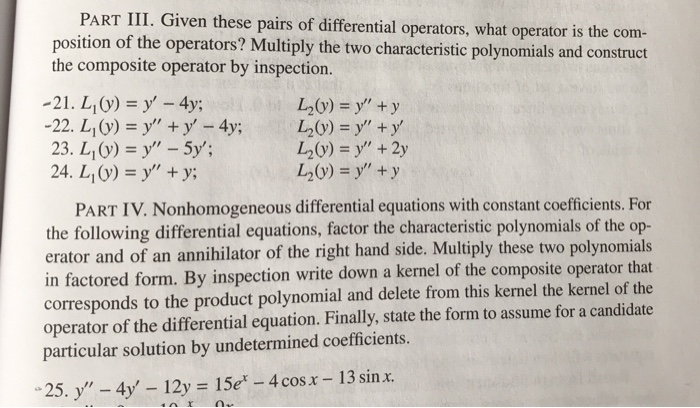Do just the marked ones (21,22,25) PART III. Given these pairs of differential operators, what operator is the com- sition of the operators? Multiply the two characteristic polynomials and construct the composite operator by inspection. L20) y" y 23. L1()" - 5y 24. Li (y) = y" + y; L2() +2y L2(y) = y" + y PART IV. Nonhomogeneous differential equations with constant coefficients. For the following differential equations, factor the characteristic polynomials of the op- erator and of an...

Free Homework App